ISSAC 94 - Symbolic-Numeric Nonlinear Equation Solving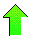Contents News View

 Two Dimensional Systems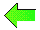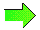Let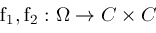be holomorphic on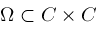;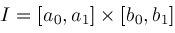be a closed real parallelepiped contained in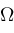;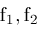be real valued on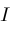; curves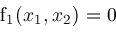and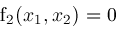intersect in a finite number of points; the partial derivatives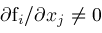at each such point; the Jacobian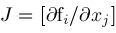is nonsingular at each such point.

The two dimensional algorithm is like the one dimensional algorithm in that it uses bisection.  However in this case, bisection means cutting rectangles in half, either widthwise or lengthwise depending on which way is most profitable in improving precision.  After each bisection,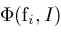and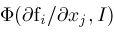are computed.  If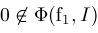or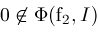, thencan be eliminated.  Otherwise, continued bisection producessuch that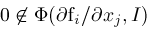, in which case the extrema of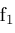and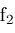must occur at the corners of.  It is then possible to determine if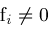on some edge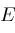ofand if so to use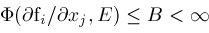to perform narrowing.

Bisection and narrowing ultimately produce a parallelepipedsuch that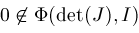and bothandchange sign on.  A nonsingular Jacobian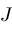implies thatandcan intersect in at most one point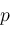in.  Each curve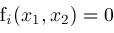intersects the boundary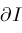oftwice at points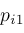and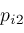.  Supposingis in the interior of, then all 4 intersection points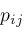are distinct and can be isolated from each other.  If the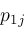points alternate with the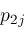points around the perimeter of, then the two curves actually do intersect and pointexists.  Otherwise, the two curves do not intersect anddoes not exist.

The generalization of Newton's formula to two dimensions is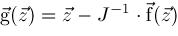and bounds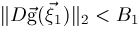and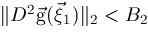must be determined.